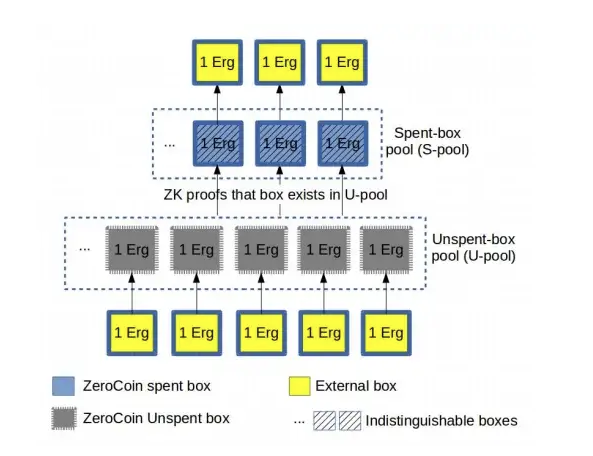# Exploring ZeroJoin#

Mixers are crucial tools for restoring fungibility in digital tokens. In Ergo, ZeroJoin is the first such mechanism implemented. It's built on the principles of ring signatures and a proof of knowledge for a Diffie-Hellman tuple, defined as (for publicly known g, h, u, v, there exists w, such as g&w == u and hw == v).

ZeroJoin utilizes two-party interactions known as Σ-protocols. We focus on two distinct types of Σ-protocols as explained below.

Let's consider G as a multiplicative group of prime order q, where the Decision Diffie-Hellman (DDH) problem is hard.

1. The first protocol, termed as proveDlog(u), is a proof of knowledge of the Discrete Logarithm of a specific group element u in relation to a fixed generator g. Essentially, the prover confirms the knowledge of x such that u = gx, using Schnorr signatures.

2. The second protocol primitive, denoted as proveDHTuple(g, h, u, v), shows proof of knowledge of a Diffie-Hellman Tuple. In this case, the prover confirms the knowledge of x such that u = gx and v = hx for arbitrary generators g and h.

This second protocol essentially executes two instances of the first protocol simultaneously.

The protocol operates as follows:

1. The prover randomly selects r from Zq, computes (t0, t1) = (gr , hr), and sends (t0, t1) to the verifier.
2. The verifier randomly picks c from Zq and sends c to the prover.
3. The prover then forwards z = r + cx to the verifier. The verifier accepts if gz equals t0 · uc and hz equals t1 · vc.

For non-interactive applications, we employ a variant of the protocol obtained through a Fiat-Shamir transformation. In this case, c equals H(t0‖t1‖m) for a specific message m to be signed.

It's important to note that the verification of proveDHTuple requires four exponentiations, while proveDlog only needs two.

Both protocols are supported by ErgoScript, offering the essential components for implementing ZeroJoin.For more details on ZeroJoin, please refer to the ZeroJoin Presentation.